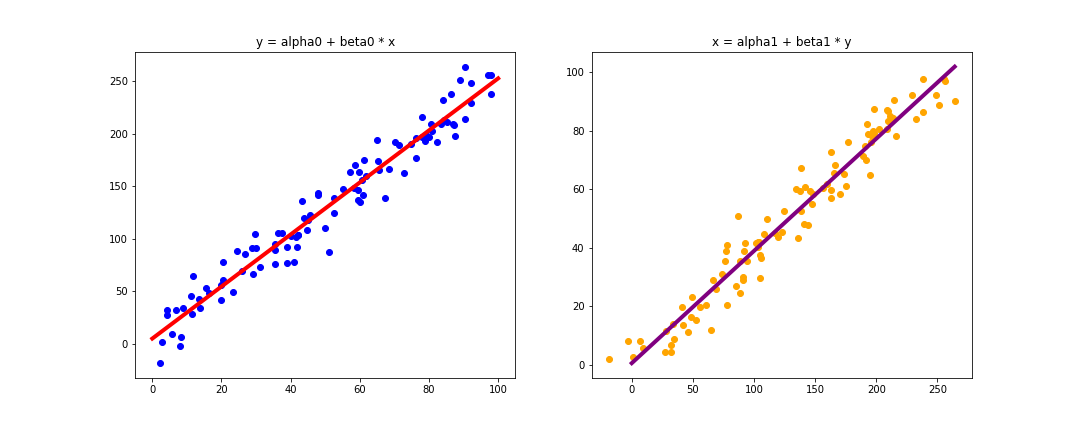### Intro to Data Science

Week 5: Intro to Linear Models

October 1, 2018

###### Today's Agenda
1. Linear Regression
2. Assumptions for Linear Models
3. Measuring Performance for Linear Models
4. Regression vs. Classification
5. Logistic Regression
###### Week 4 Recap
1. Types of Data
2. Useful Statistical Distribution
3. Important Summary Statistics
4. Key Theorems
###### HW Recap
1. How was DataCamp?
• What are the key relationships from the data sets you've selected?
• How might you apply a model to your data?
###### Data Science ModelsWhat is linear regression?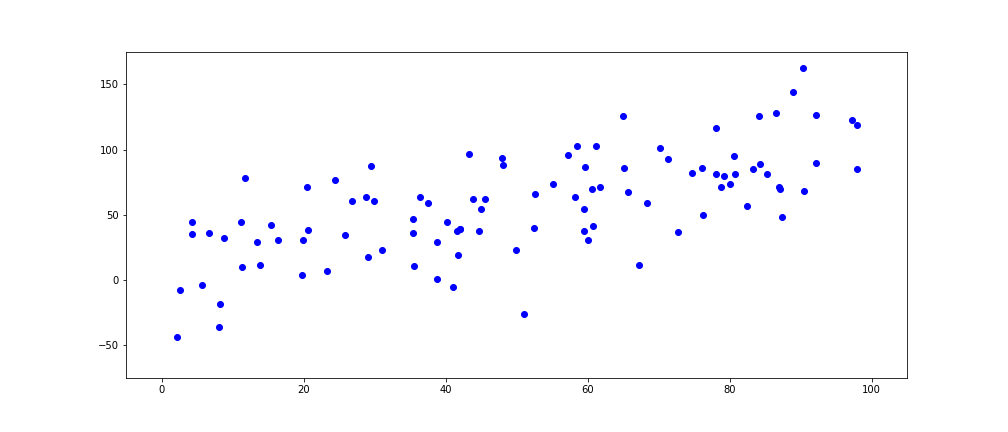What is linear regression?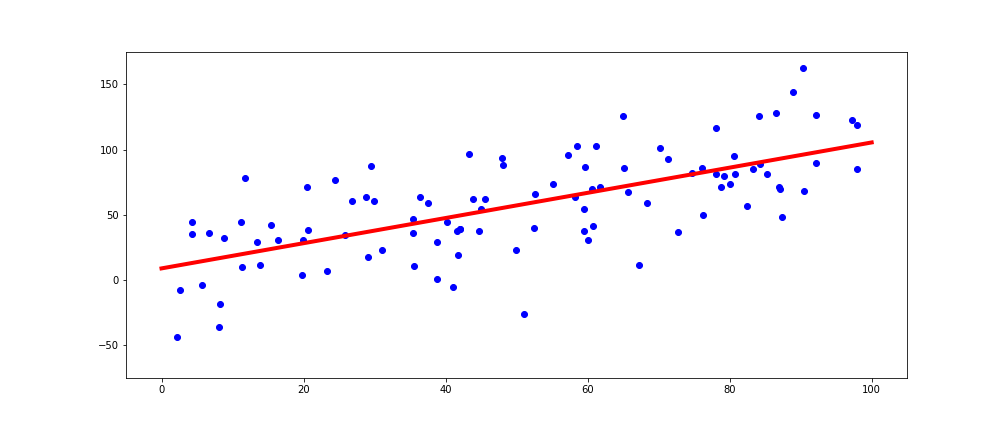Why do we call this a linear regression?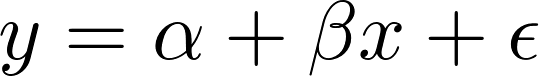A model is linear when each term is either a constant or the product of a parameter and a predictor variable.

What are we trying to solve for?Demo
###### Assumptions of Linear Regression
1. Data is linear in form.
2. Sample is random.
3. Error terms have constant variance (homoscedasticity).
4. Error terms have a mean of zero based on the observed data.
5. Predictors are independent (no multicollinearity).
6. Errors are normally distributed.
###### Data is linear in form.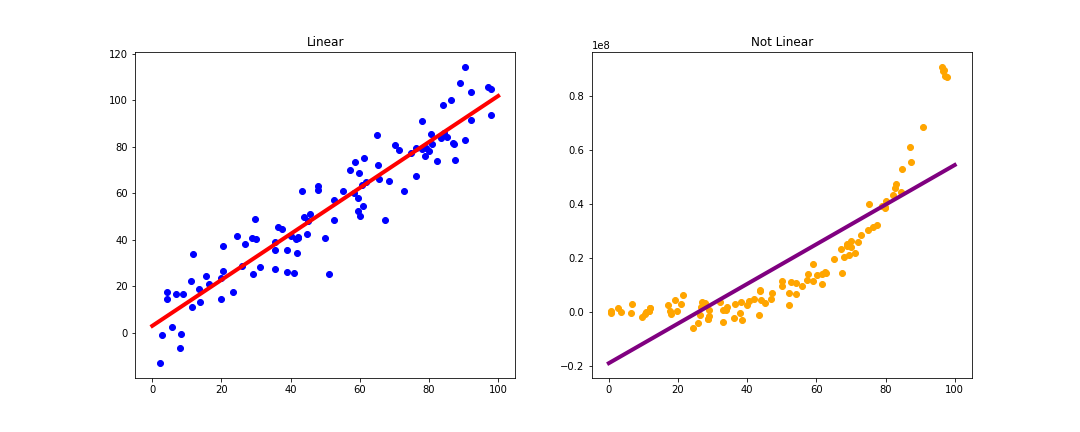###### Sample is random.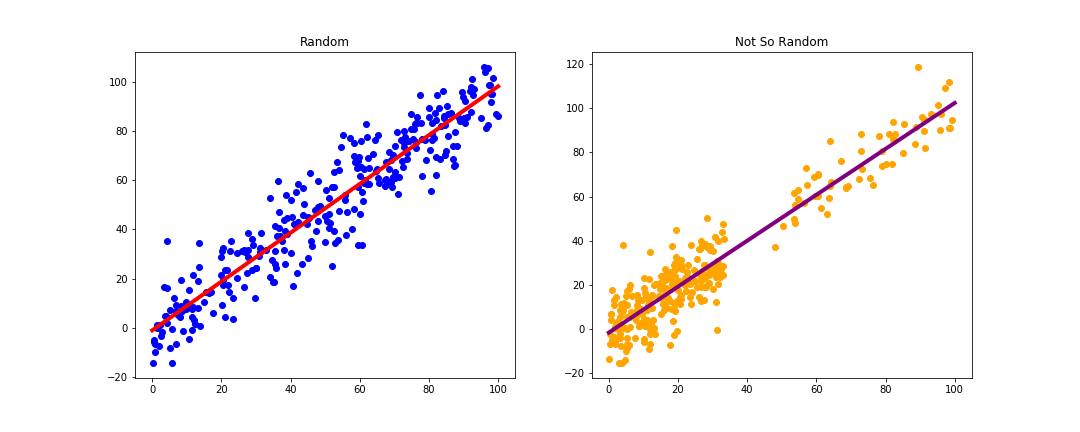###### Error terms have constant variance (homoskedasticity).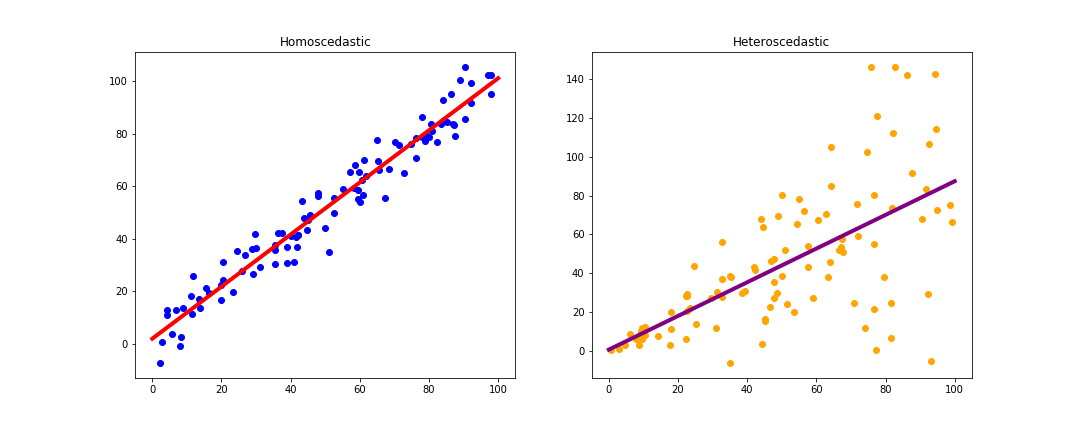###### Errors are uncorrelated.###### Predictors are independent (no multicollinearity).

in the case of: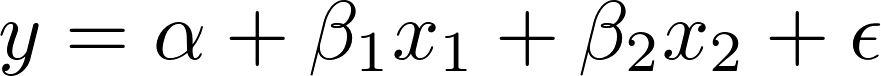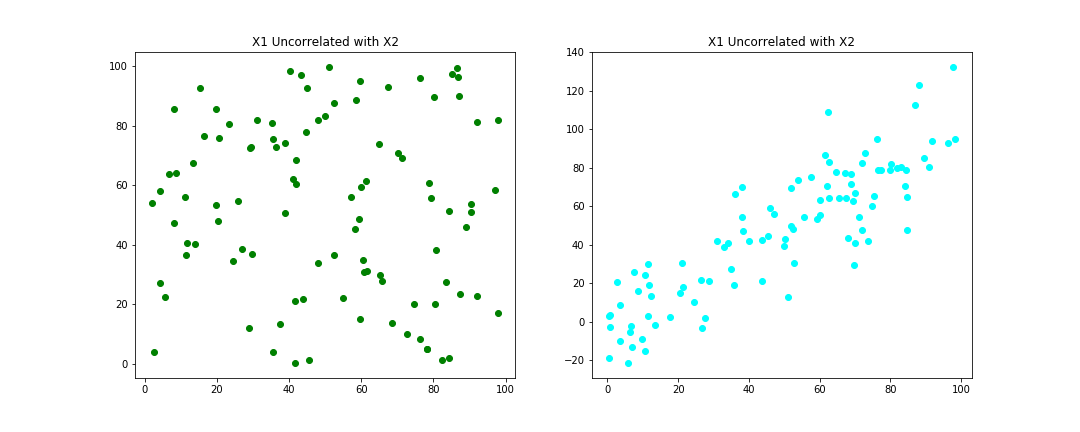###### Errors are normally distributed.###### Output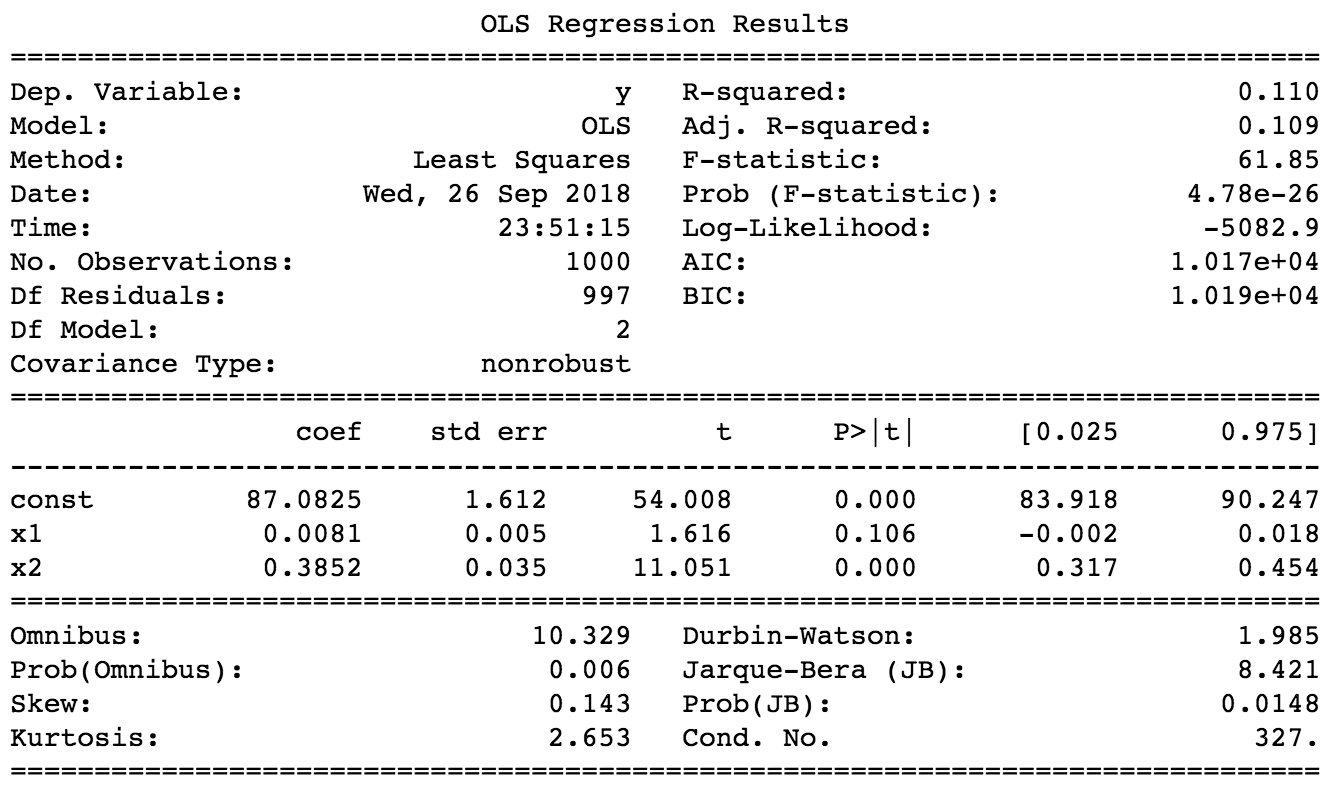###### Key Metrics of Success
1. R-squared
3. Coefficients
4. P-values
###### R-Squared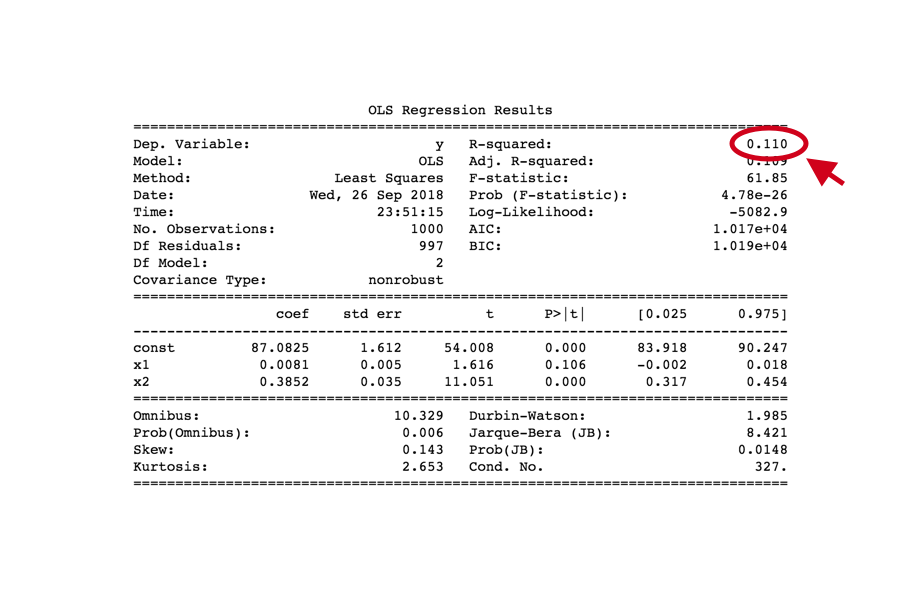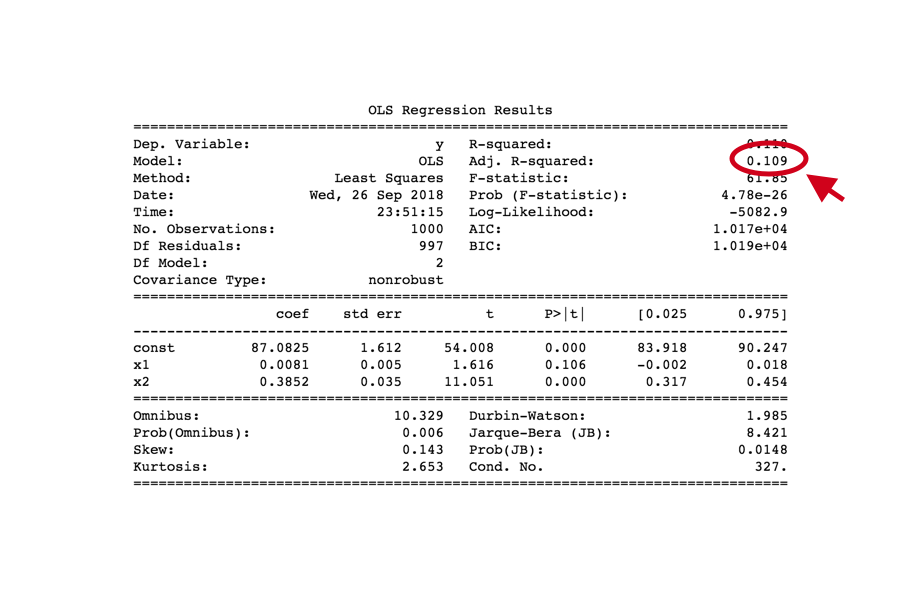###### Coefficients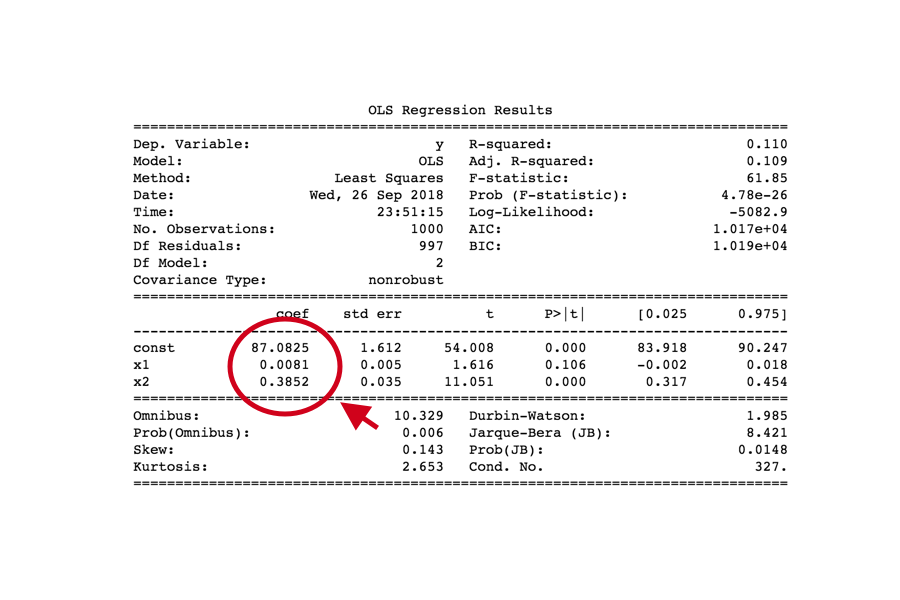###### P-values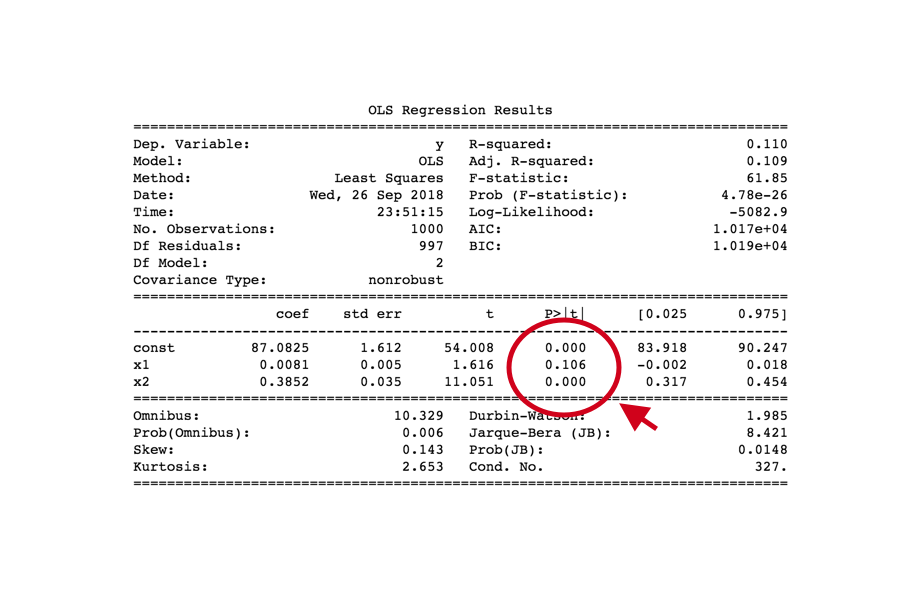xkcd
###### Does linear regression prove causality?